Wizard sizing / Machine information

Machine information

The Machine information regarding the selected application is input.
In the case of load the data with Data load / delete and input them in that time. When data were load with Data load/ delete, or Machine Infomatino already inputted, the Machine Infomation that were already finished setting are displayed.

[Operation Procedure]

1. Input data in the data input column.
The saved data is loaded if necessary (Click the Load data button).
The Inertia calculation can calculate the inertia value.
The Transmission Calculate button is displayed beside the Gear Ratio input column.
Because this advances to Transmission Calculate when you click this button, you can calculate Gear ratio by setting Transmission.
The Inertia Calculate button is displayed beside the Inertia input column.
Because this advances to Inertia Calculate when you click this button, you can calculate Inertia.
2. Click "Next" to advance to the Velocity diagram.

*Relations between movement direction of load and signs of velocity
Here defines that right direction of the screen and upper direction of the movement of a load as "plus".
In other word, when you input a plus value at speed parameter with Velocity diagram, a load moves to right direction or upper direction.
Or, the external force of a load is defined a external force from a right direction or a upper direction as plus, and is defined a external force from a left direction or a lower direction as minus.

*Relations between total efficiency and gear efficiency
Total efficiency whole of mechanism dose not included gear efficiency.
If you want to input efficiency of whole machine, you need to input it at the column of total efficiency, and to input 1 at the column of gear efficiency.

[Screen Structure]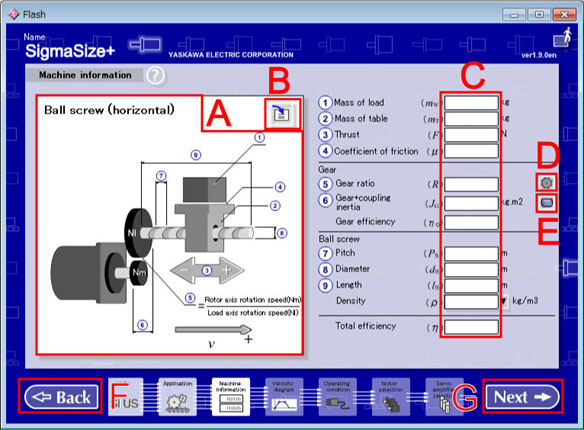Machine information Screen (Ball Screw <horizontal>)

1. Application Preview
A selected application is zoomed in.

2. Load Data button
The value of the Machine information is loaded from saved data.
The data loaded is converted to the current set unit.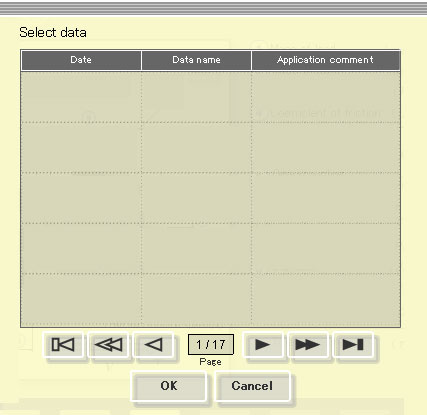1. Data list
Data is displayed from the latest one at the date.
Click and select the following page button if necessary.

2. Select the data loaded then click "OK".
Goes to the previous screen without loading when click a "Cancel".

3. Data Input Column
In init state, all columns are blank.
When data is read or hold, the value at that time is displayed.

The number of maximum input beams of each input column is 12 figures(include such as a mark and a decimal point).
Example
Possible  :1.0,-1.0,1.2345678912,-1.234567891,1.0e+100  etc.
Impossible;:1.00000000000,-1000000000,1.23456789123,1.000000e+001  etc.

 Rotary type Ball screw (horizontal) Timing belt (horizontal) Rack & pinion (horizontal) Ball screw (vertical) Timing belt (vertical) Rack & pinion (vertical) Roll feeder Rotor Rotation table Linear type Linear single axis

4. Transmission calculation button
The Transmission calculation window is open.
*Displays beside the gear ratio input column.

5. Inertia calculation button
The Inertia calculation window is open.
*Displays beside the inertia input column.

6. Back button
Returns to the Application.

7. Next button
Advances to the Velocity diagram.

## [Mechanism Parameter and Equation in each application]

 Constants Gravity g Circular constant π Input without Mechanism parameter Rotary type motor Rotor moment of ienrtia JM Motor option moment of inertia JMO Linear type motor Moving coil mass mM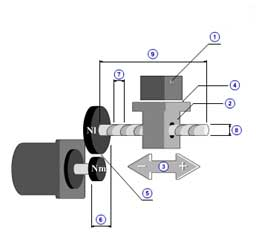1 Mass of load( mW ) 2 Mass of table( mT ) 3 Thrust( F ) 4 Coefficient of friction( μ ) 5 Gear ratio( R ) 6 Gear + coupling inertia( JG ) Gear efficiency( ηG ) 7 Pitch( PB ) 8 Diameter( dB ) 9 Length( lB ) Density( ρ ) Total efficiency( η )

 Frictional force FFR = μ ( mW + mT )g (N) Constant external force FC = F (N) Moment of inertia component Mechanism moment of inertia(Ball screw) JMC = πρB lB dB4 / ( 32 R 2 ) (kg.m2) Load mass moment of inertia JW = (mW + mT )( Kvn / 2π )2 (kg.m2) Load moment of inertia JL = JMC + JG + JW (kg.m2) Total load moment of inertia JA = JL + JM + JMO (kg.m2) * Kvn = PB / R (m/rev) Torque component Torque by friction TFR = FFR Kvn / ( 2π ) (N.m) Constant torque TC = FC  Kvn / ( 2π ) (N.m) Torque by force from forward TFW = 0 (N.m) Torque by force from backward TBW = 0 (N.m)

Required torque

[Back]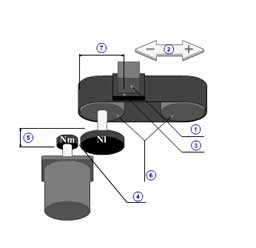1 Mass of load( mW ) 2 Thrust( F ) 3 Coefficient of friction( μ ) 4 Gear ratio( R ) 5 Gear + coupling inertia( JG ) Gear efficiency( ηG ) 6 Pulley inertia( JP ) 7 Pulley diameter( dP ) Total efficiency( η )
 Friction force FFR = μ mW g (N) Constant external force FC = F (N) Momemnt of inertia component Mechanism moment of inertia(Pulley) JMC = JP / R 2 (kg.m2) Load mass moment of inertia JW = mW ( Kvn / 2π )2 (kg.m2) Load moment of inertia JL = JMC + JG + JW (kg.m2) Total load moment of inertia JA = JL + JM + JMO (kg.m2) * Kvn = π dP / R (m/rev) Torque component Torque by friction TFR = FFR Kvn / ( 2π ) (N.m) Consntant torque TC = FC  Kvn / ( 2π ) (N.m) Torque by force from forward TFW = 0 (N.m) Torque by force from backward TBW = 0 (N.m)

Required torque

[Back]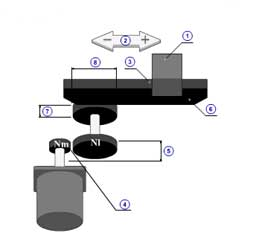1 Mass of load( mW ) 2 Thrust( F ) 3 Coefficient of friction( μ ) 4 Gear ratio( R ) 5 Gear + coupling inertia( JG ) Gear efficiency( ηG ) 6 Mass of rack( mR ) 7 Pinion inertia( JPIN ) 8 Pinion diameter( dPIN ) Total efficiency( η )

 Frictional force FFR = μ ( mW + mR )g (N) Consntant external force FC = F (N) Moment of inertia component Mechanism moment of inertia(Pinion) JMC = JPIN / R 2 (kg.m2) Load mass moment of inertia JW = ( mW + mR )( Kvn / 2π )2 (kg.m2) Load moment of inertia JL = JMC + JG + JW (kg.m2) Total load moment of inertia JA = JL + JM + JMO (kg.m2) * Kvn = π dPIN / R (m/rev) Torque component Torque by friction TFR = FFR Kvn / ( 2π ) (N.m) Constant torque TC = FC  Kvn / ( 2π ) (N.m) Torque by force from forward TFW = 0 (N.m) Torque by force from backward TBW = 0 (N.m)

Required torque

[Back]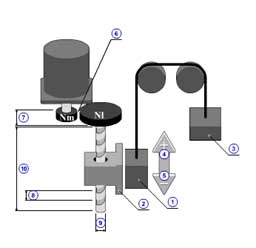1 Mass of load( mW ) 2 Mass of table( mT ) 3 Mass of counter( mWC ) 4 Thrust in ascending( FVU ) 5 Thrust in descending( FVD ) 6 Gear ratio( R ) 7 Gear + coupling inertia( JG ) Gear efficiency( ηG ) 8 Pitch( PB ) 9 Diameter( dB ) 10 Length( lB ) Density( ρ ) Total efficiency( η ) *Reference When you consider the resistance that a load is moving in a lower direction. Input a plus value in the Thrust in descending column. When you consider the resistance that a load is moving in a upper direction. Input a minus value in the Thrust in ascending column.

 Frictional force FFR = 0 (N) Constant external force(Thrust + Gravity) FC = - ( mW + mT - mWC )g (N) Force from forward FFW = FVU (N) Force from backward FBW = FVD (N) Moment of inertia component Mechanism moment of inertia(Ball screw) JMC = π ρB lB dB4 / ( 32 R 2 ) (kg.m2) Load mass moment of inertia JW = ( mW + mT + mWC )( Kvn / 2π )2 (kg.m2) Load moment of inertia JL = JMC + JG + JW (kg.m2) Total load moment of inertia JA = JL + JM + JMO (kg.m2) * Kvn = PB / R (m/rev) Torque component Torque by friction TFR = FFR Kvn / ( 2π ) (N.m) Constant torque TC = FC  Kvn / ( 2π ) (N.m) Torque by force from forward TFW = FFW Kvn / ( 2π ) (N.m) Torque by force from backward TBW = FBW Kvn / ( 2π ) (N.m)

Required torque

[Back]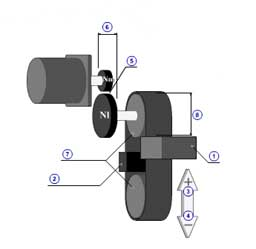1 Mass of load( mW ) 2 Mass of counter( mWC ) 3 Thrust in ascending( FVU ) 4 Thrust in descending( FVD ) 5 Gear ratio( R ) 6 Gear + coupling inertia( JG ) Gear efficiency( ηG ) 7 Pulley inertia( JP ) 8 Pulley diameter( dP ) Total efficiency( η )

 Frictional force FFR = 0 (N) Constant external force(Thrust+Gravity) FC = - ( mW + mT - mWC )g (N) Torque by force from forward FFW = FVU (N) Torque by force from backward FBW = FVD (N) Moment of inertia component Mechanism moment of inertia(Pulley) JMC = π ρB lB dB4 / ( 32 R 2 ) (kg.m2) Load mass moment of inertia JW = ( mW + mT + mWC )( Kvn / 2π )2 (kg.m2) Load moment of inertia JL = JMC + JG + JW (kg.m2) Total load moment of inertia JA = JL + JM + JMO (kg.m2) * Kvn = PB / R (m/rev) Torque component Torque by friction TFR = FFR Kvn / ( 2π ) (N.m) Constant torque TC = FC  Kvn / ( 2π ) (N.m) Torque by force from forward TFW = FFW Kvn / ( 2π ) (N.m) Torque by force from backward TBW = FBW Kvn / ( 2π ) (N.m)

Required torque

[Back]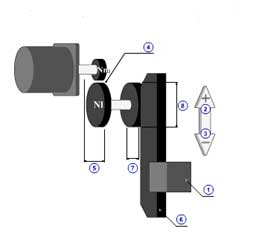1 Mass of load( mW ) 2 Thrust in ascending( FVU ) 3 Thrust in descending( FVD ) 4 Gear ratio( R ) 5 Gear + coupling inertia( JG ) Gear efficiency( ηG ) 6 Mass of rack( mR ) 7 Pinion inertia( JPIN ) 8 Pinion diameter( dPIN ) Total efficiency( η )

 Frictional force FFR = 0 (N) Constant force(Thrust+gravity) FC = - ( mW + mR )g (N) Force from forward FFW = FVU (N) Force from backward FBW = FVD (N) Moment of inertia component Mechanism moment of inertia(Pinion) JMC = JPIN / R 2 (kg.m2) Load mass moment of inertia JW = ( mW + mR )( Kvn / 2π )2 (kg.m2) Load moment of inertia JL = JMC + JG + JW (kg.m2) Total load moment of inertia JA = JL + JM + JMO (kg.m2) * Kvn = π dPIN / R (m/rev) Torque component Torque by friction TFR = FFR Kvn / ( 2π ) (N.m) Constant torque TC = FC  Kvn / ( 2π ) (N.m) Torque by force from forward TFW = FFW Kvn / ( 2π ) (N.m) Torque by force from backward TBW = FBW Kvn / ( 2π ) (N.m)

Required torque

[Back]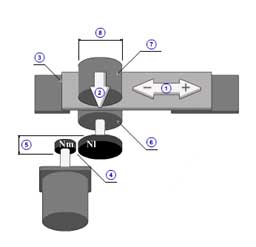1 Tension( F ) 2 Press( FP ) 3 Coefficient of friction( μ ) 4 Gear ratio( R ) 5 Gear + coupling inertia( JG ) Gear efficiency( ηG ) 6 Driving roller inertia( JR1 ) 7 Driven roller inertia( JR2 ) 8 Driving roller diameter( dR ) Total efficiency( η ) *Reference when the movement direction of a load is a right direction and the tension against a load is a left direction. Input a plus value in the speed(v) column with Velocity diagram and a minus value in the Tension(F) column. when driven roller's diameter and driving roller's diameter are different. when there are a driven roller's inertia J'R2 and its diameter dR2 , driven roller inertia JR2 is indicated as follows. JR2 = J'R2 ( dR / dR2 )2

 Frictional force FFR = μ FP (N) Constant external force FC = F (N) Moment of inertia component Mechanism moment of inertia(Roller) JMC = ( JR1 + JR2 ) / R 2 (kg.m2) Load mass moment of inertia JW = 0 (kg.m2) Load moment of inertia JL = JMC + JG + JW (kg.m2) Total load moment of inertia JA = JL + JM + JMO (kg.m2) * Kvn = π dR / R (m/rev) Torque component Torque by friction TFR = FFR Kvn / ( 2π ) (N.m) Constant torque TC = FC  Kvn / ( 2π ) (N.m) Torque by force from forward TFW = 0 (N.m) Torque by force from backward TBW = 0 (N.m)

Required torque

[Back]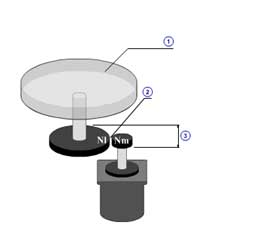1 Load inertia( JWL ) Friction torque( TFL ) 2 Gear ratio( R ) 3 Gear + coupling inertia( JG ) Gear efficiency( ηG ) Total efficiency( η )

 Mechanism moment of inertia(Rotor) JMC = JWL / R 2 (kg.m2) Load mass moment of inertia JW = 0 (kg.m2) Moment of inertia component Load moment of inertia JL = JMC + JG + JW (kg.m2) Total load moment of inertia JA = JL + JM + JMO (kg.m2) * Kvn = 1 / R Torque component Torque by friction TFR = TFL Kvn (N.m) Consntant torque TC = 0 (N.m) Torque by force from forward TFW = 0 (N.m) Torque by force from backward TBW = 0 (N.m)

Required torque

[Back]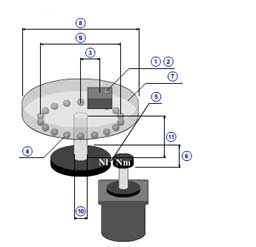1 Load inertia( JWL ) 2 Mass of load( mWL ) 3 Radius of load mass( rWL ) 4 Coefficient of friction( μ ) 5 Gear ratio( R ) 6 Gear + coupling inertia( JG ) Gear efficiency( ηG ) 7 Mass of rotation table( mT ) 8 Rotation table diameter( dT ) 9 Holding shaft diameter( dF ) 10 Main shaft diameter( dS ) 11 Main shaft length( lS ) Main shaft density( ρS ) Total efficiency( η )

 Mechanism moment of inertia(Table + shaft) JMC = (π ρS lS dS4 / 32 + mT dT2 / 8 ) / R 2 (kg.m2) Load mass moment of inertia JW = ( JWL + mWL rWL2 ) / R 2 (kg.m2) Moment of inertia component Load moment of inertia JL = JMC + JG + JW (kg.m2) Total load moment of inertia JA = JL + JM + JMO (kg.m2) * Kvn = 1 / R Torque component Torque by friction TFR = μ ( mWL + mT )g ( dF / 2 ) Kvn (N.m) Constant torque TC = 0 (N.m) Torque by force from forward TFW = 0 (N.m) Torque by froce from backward TBW = 0 (N.m)

Required torque

[Back]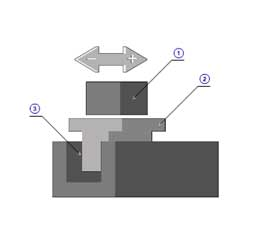1 Mass of load( mW ) 2 Mass of table( mT ) 3 Friction force( FFR ) Force margin( KM ) Total efficiency( η ) * Friction force( FFR ) Please include the following thrust by which coefficient of friction is considered to the friction force. 1.The load required thrust by which the friction coefficient of machine part is considered, such as a linear guide. 2.Mass of linear motor movable required thrust by which the friction coefficient of machine part is considered, such as a linear guide. 3.The power-of-absorption part required thrust of the linear motor with core by which the friction coefficient of machine part is considered, such as linear guide. * Force margin( KM ) This is a coefficient assuming the case which increases required force to accelerate or decelerate, on the structure of a machine. Input 1 or more value by the inertia between a moving coil and the center of gravity of load. Force to accelerate or decelerate is calculated as F = KM m a.

 Mass of load mL = mW + mT (kg) Total mass of load mA = mL + mM (kg)

Required Force

[Back]

Required Torque/Force Equation

When you set speed such as chart below, there are equations of required torque and force.
In addition, It replace speed(vi) with rotate speed(ni) in the case of rotor or rotary table.

Velocity diagram

 Section speed pattern i = 1 .. n Section time ti (s) Section speed vi (m/s)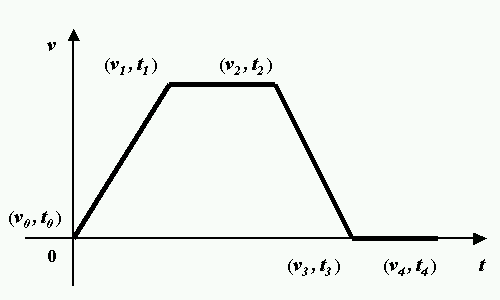Example for velocity diagram

Required torque (Rotary motor case)

 Section rotation speed difference nMdi = ( vi - vi-1 ) / Kvn (s-1) Section acceleration torque TAi = JA ( 2π nMdi / ( ti - ti-1 ) ) (N.m) Section torque Ti (N.m) Normal ( nMi > 0 OR nMi = 0, nMi-1 > 0 ) Ti = TAi - TC - TFW + TFR (N.m) Reverse ( nMi < 0 OR nMi = 0, nMi-1 < 0 ) Ti = TAi - TC - TBW - TFR (N.m) Stopping ( nMi = 0) | TC | > TFR TC > 0 Ti = - TC + TFR (N.m) TC < 0 Ti = - TC - TFR (N.m) | TC | <= TFR Ti = 0 (N.m) Effective torque TRMS = √( ∑ ni=1 Ti 2 ( ti - ti-1 ) / tn ) (N.m) Required force(Linear motor type) Section acceleration force FAi = KM mA ( vi - vi-1 ) / ( ti - ti-1 ) (N) Section force Fi (N) Normal (vi > 0 OR vi = 0, vi-1 > 0 ) Fi = FAi + (   FFR / η ) (N) Reverse (vi < 0 OR vi = 0, vi-1 < 0 ) Fi = FAi + ( - FFR / η ) (N) Stopping (vi = 0, vi = 0 ) Fi = 0 (N) Effective force FRMS = √( ∑ ni=1 Fi 2 ( ti - ti-1) / tn ) (N)

[
Back]